SPSS TUTORIALS BASICS DATA ANALYSIS REGRESSION ANOVA T-TESTS

# Creating Dummy Variables in SPSS

You can't readily use categorical variables as predictors in linear regression: you need to break them up into dichotomous variables known as dummy variables.

The ideal way to create these is our dummy variables tool. If you don't want to use this tool, then this tutorial shows the right way to do it manually.

## Example Data File

This tutorial uses staff.sav throughout. Part of this data file is shown below.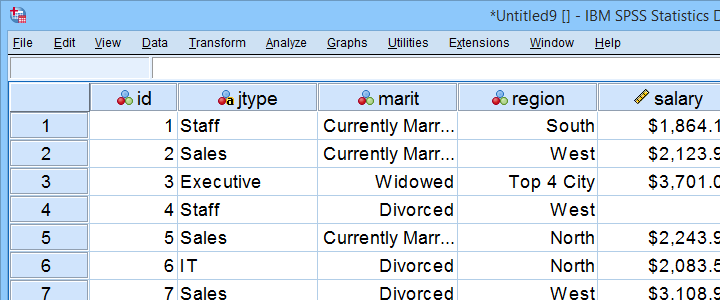## Example I - Any Numeric Variable

Let's first create dummy variables for marit, short for marital status. Our first step is to run a basic FREQUENCIES table with frequencies marit. The table below shows the resulting table.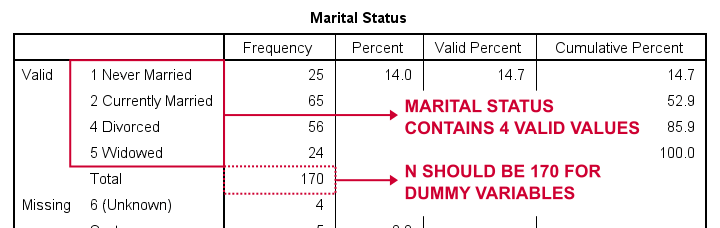So how to break up marital status into dummy variables? First off, we always omit one category, the reference category. You may choose any category as the reference category.

So for this example, we choose 5 (Widowed). This implies that we'll create 3 dummy variables representing categories 1, 2 and 4 (note that 3 does not occur in this variable).

The syntax below shows how to create and label our 3 dummy variables. Let's run it.

*Create dummy variables for categories 1, 2 and 4.

compute marit_1 = (marit = 1).
compute marit_2 = (marit = 2).
compute marit_4 = (marit = 4).

*Apply variable labels to dummy variables.

variable labels
marit_1 'Marital Status = Never Married'
marit_2 'Marital Status = Currently Married'
marit_4 'Marital Status = Divorced'.

*Quick check first dummy variable

frequencies marit_1.

## Results

First off, note that we created 3 nicely labelled dummy variables in our active dataset.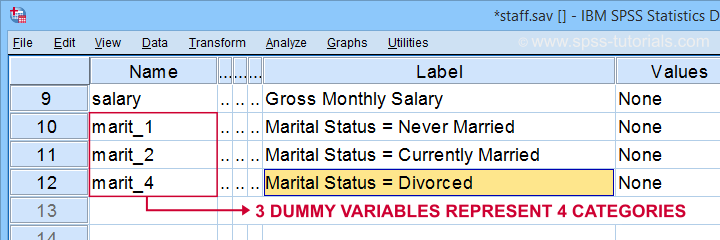The table below shows the frequency distribution for our first dummy variable.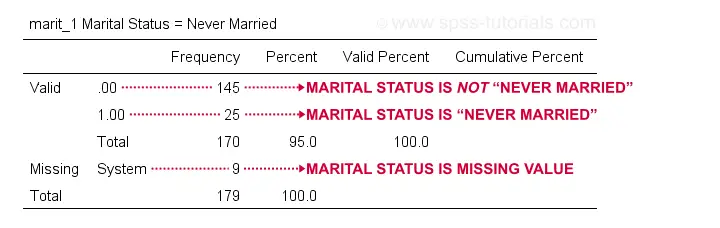Note that our dummy variable holds 3 distinct values:

• respondents whose marital status is not “never married” score 0;
• respondents whose marital status is “never married” score 1;
• respondents whose marital status is a missing value (and therefore unknown) have a system missing value.

We may now check the results more thoroughly by running crosstabs marit by marit_1 to marit_4. Doing so creates 3 contingency tables, the first of which is shown below.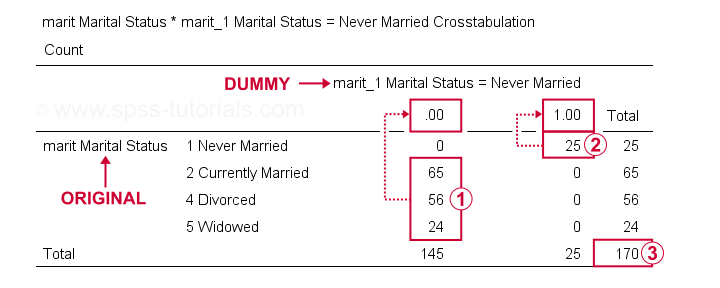On our dummy variable,respondents having other marital statuses than “never married” all score 0;respondents who “never married” all score 1;we've a sample size of N = 170 (this table only includes respondents without missing values on either variable).

Optionally, a final -very thorough- check is to compare ANOVA results for the original variable to regression results using our dummy variables. The syntax below does just that, using monthly salary as the dependent variable.

*Minimal regression using dummy variables.

regression
/dependent salary
/method enter marit_1 to marit_4.

*Minimal ANOVA using original variable.

oneway salary by marit.

Note that both analyses result in identical ANOVA tables. We'll discuss ANOVA versus dummy variable regression more thoroughly in a future tutorial.

## Example II - Numeric Variable with Adjacent Integers

We'll now create dummy variables for region. Again, we start off by inspecting a minimal frequency table which we'll create by running frequencies region. This results in the table below.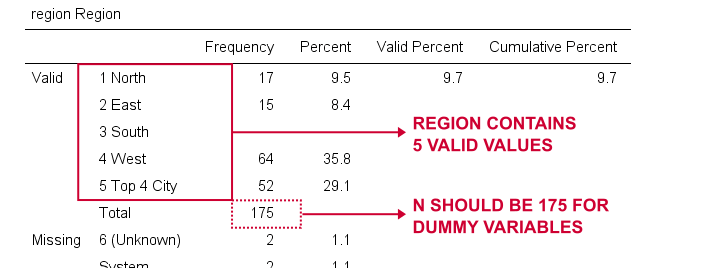We'll choose 1 (“North”) as our reference category. We'll therefore create dummy variables for categories 2 through 5. Since these are adjacent integers, we can speed things up by using DO REPEAT as shown below.

*Create dummy variables for region categories 2 through 5.

do repeat #vals = 2 to 5 / #vars = region_2 to region_5.
recode region (#vals = 1)(lo thru hi = 0) into #vars.
end repeat print.

*Apply variable labels to new variables.

variable labels
region_2 'Region = East'
region_3 'Region = South'
region_4 'Region = West'
region_5 'Region = Top 4 City'.

*Quick check.

crosstabs region by region_2 to region_5.

A careful inspection of the resulting tables confirms that all results are correct.

## Example III - String Variable with Conversion

Sadly, our first 2 methods don't work for string variables such as jtype -short for “job type”). The easiest solution is to convert it into a numeric variable as discussed in SPSS Convert String to Numeric Variable. The syntax below uses AUTORECODE to get the job done.

*Convert jtype into numeric variable.

autorecode jtype
/into njtype.

*Check result.

frequencies njtype.

*Set missing values.

missing values njtype (1,2).

*Recheck result.

frequencies njtype.

## Result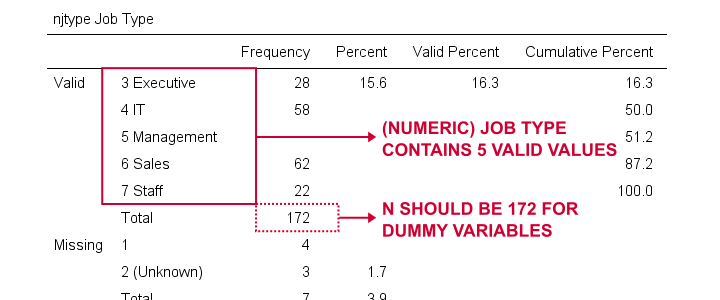Since njtype -short for “numeric job type”- is a numeric variable, we can now use method I or method II for breaking it up into dummy variables.

## Example IV - String Variable without Conversion

Converting string variables into numeric ones is the easy to create dummy variables for them. Without this conversion, the process is cumbersome because SPSS doesn't handle missing values for string variables properly. However, syntax below gets the job done correctly.

*Inspect frequencies.

frequencies jtype.

*Chance '(Unknown)' into 'NA'.

recode jtype ('(Unknown)' = 'NA').

*Set user missing values.

missing values jtype ('','NA').

*Reinspect frequencies.

frequencies jtype.

*Create dummy variables for string variable.

if(not missing(jtype)) jtype_1 = (jtype = 'IT').
if(not missing(jtype)) jtype_2 = (jtype = 'Management').
if(not missing(jtype)) jtype_3 = (jtype = 'Sales').
if(not missing(jtype)) jtype_4 = (jtype = 'Staff').

*Apply variable labels to dummy variables.

variable labels
jtype_1 'Job type = IT'
jtype_2 'Job type = Management'
jtype_3 'Job type = Sales'
jtype_4 'Job type = Staff'.

*Check results.

crosstabs jtype by jtype_1 to jtype_4.

## Final Notes

Creating dummy variables for numeric variables can be done fast and easily. Setting proper variable labels, however, always takes a bit of work. String variables require some extra step(s) but are pretty doable as well.

Nevertheless, the easiest option is our SPSS Create Dummy Variables Tool as it takes perfect care of everything.

Hope you found this tutorial helpful! Let us know by throwing a comment below.

Thanks for reading!

# Tell us what you think!

*Required field. Your comment will show up after approval from a moderator.